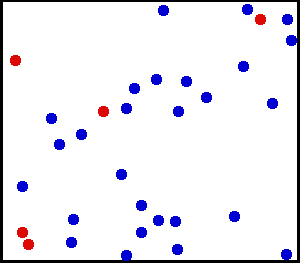## 引擎

$\mathbf{r}(t) = \mathbf{p} + \mathbf{v}*t$

$\left | \mathbf{r_1}(t)-\mathbf{r_2}(t) \right |=2R$

$\begin{matrix} \mathbf{v_1'}& = & \mathbf{v_1}-\frac{2m_2}{m_1+m_2} \frac{\left \langle \mathbf{v_1}-\mathbf{v_2},\mathbf{x_1}-\mathbf{x_2} \right \rangle}{\left \| \mathbf{x_1}-\mathbf{x_2} \right \|^2}(\mathbf{x_1}-\mathbf{x_2})\\ \mathbf{v_2'}& = & \mathbf{v_2}-\frac{2m_1}{m_1+m_2} \frac{\left \langle \mathbf{v_1}-\mathbf{v_2},\mathbf{x_1}-\mathbf{x_2} \right \rangle}{\left \| \mathbf{x_2}-\mathbf{x_1} \right \|^2}(\mathbf{x_2}-\mathbf{x_1}) \end{matrix}$

## 维护碰撞事件

### $O(N\log N)$

1. 将所有点的碰撞事件队列中，与xy碰撞的事件发生的时间，标记成 $+\infty$
2. 重新计算xy的整个碰撞事件队列
3. 扫一遍所有点的碰撞事件队列，找出下一次碰撞时间最近的碰撞事件，作为下一次碰撞事件